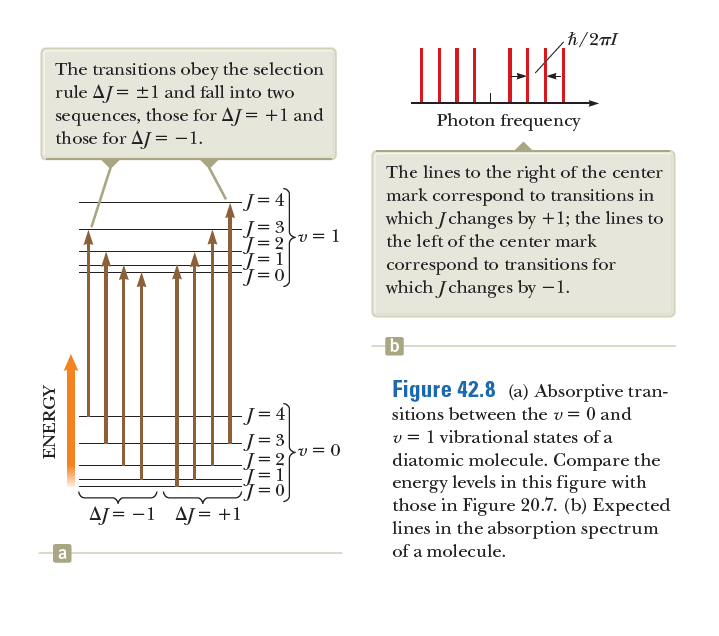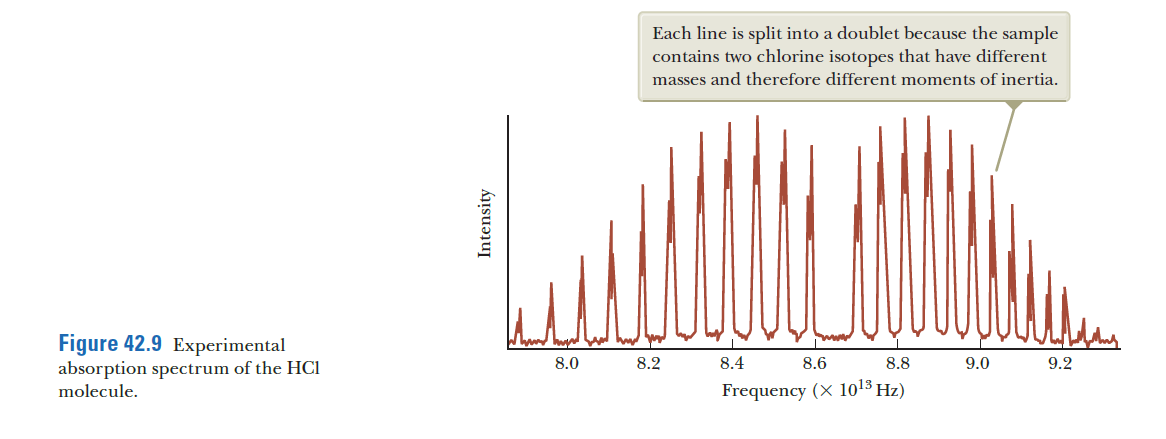# h/2nl The transitions obey the selection rule AJ = ±1 and fall into two sequences, those for AJ= +1 and those for AJ = -1. IIII. HA. Photon frequency The lines to the right of the center mark correspond to transitions in which Jchanges by +1; the lines to -J= 4 = 3 v = 1 the left of the center mark correspond to transitions for which Jchanges by –1. b Figure 42.8 (a) Absorptive tran- -J= 4 sitions between the v= 0 and v = 1 vibrational states of a diatomic molecule. Compare the energy levels in this figure with those in Figure 20.7. (b) Expected lines in the absorption spectrum -v = 0 AJ = -1 AJ= +1 a of a molecule. IL || ||| I| || || || ENERGY Each line is split into a doublet because the sample contains two chlorine isotopes that have different masses and therefore different moments of inertia. Figure 42.9 Experimental absorption spectrum of the HCI molecule. 8.0 8.2 8.4 8.6 8.8 9.0 9.2 Frequency (X 1013 Hz)

Question

In 42.8a, the transitions indicated correspond to spectral lines that are equally spaced as shown in 42.8b. The actual spectrum in 42.9, however, shows lines that move closer together as the frequency increases. Why does the spacing of the actual spectral lines differ from the diagram in 42.8b?help_outlineImage Transcriptioncloseh/2nl The transitions obey the selection rule AJ = ±1 and fall into two sequences, those for AJ= +1 and those for AJ = -1. IIII. HA. Photon frequency The lines to the right of the center mark correspond to transitions in which Jchanges by +1; the lines to -J= 4 = 3 v = 1 the left of the center mark correspond to transitions for which Jchanges by –1. b Figure 42.8 (a) Absorptive tran- -J= 4 sitions between the v= 0 and v = 1 vibrational states of a diatomic molecule. Compare the energy levels in this figure with those in Figure 20.7. (b) Expected lines in the absorption spectrum -v = 0 AJ = -1 AJ= +1 a of a molecule. IL || ||| I| || || || ENERGY fullscreenhelp_outlineImage TranscriptioncloseEach line is split into a doublet because the sample contains two chlorine isotopes that have different masses and therefore different moments of inertia. Figure 42.9 Experimental absorption spectrum of the HCI molecule. 8.0 8.2 8.4 8.6 8.8 9.0 9.2 Frequency (X 1013 Hz) fullscreen

### Want to see this answer and more?

Experts are waiting 24/7 to provide step-by-step solutions in as fast as 30 minutes!*

*Response times may vary by subject and question complexity. Median response time is 34 minutes for paid subscribers and may be longer for promotional offers.
Tagged in
Science